|

# 倾听古罗马故事 法恩莎瓷砖·臻稀石3代罗马御石评测建筑本身是没有生命的，但不少人说，建筑是有生命的，是有记忆的。古罗马斗兽场，建于公元72至82年间，是古罗马为取悦凯旋的将领士兵、赞美伟大的古罗马帝国而建造的。置身其中，我们仿佛能从断垣残壁中，从砖瓦的纹理中，看到当年伟大令人敬畏的古罗马帝国，将领士兵们在族人的欢呼喝彩声中凯旋而归，还有角斗士与野兽顽强搏斗直到一方死亡为止的惨烈与悲壮。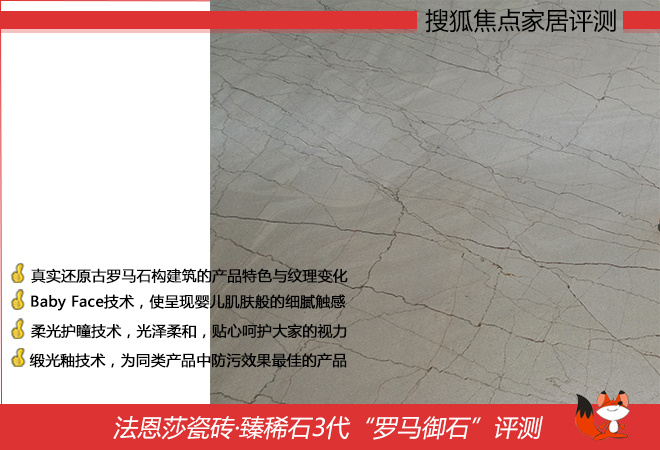今天搜狐焦点家居为评测室为大家带来的是法恩莎瓷砖臻稀石3代罗马御石，其灵感来源正是古罗马的建筑，砖面纹理纵横交错，真实还原古罗马石构建筑的产品特色与纹理变化，恢宏大气，充满历史底蕴，尊贵天成。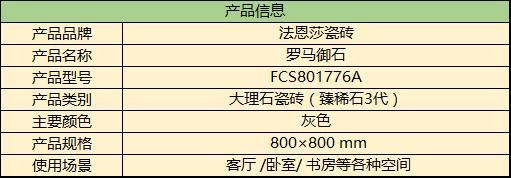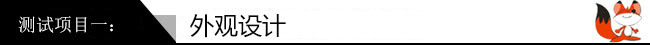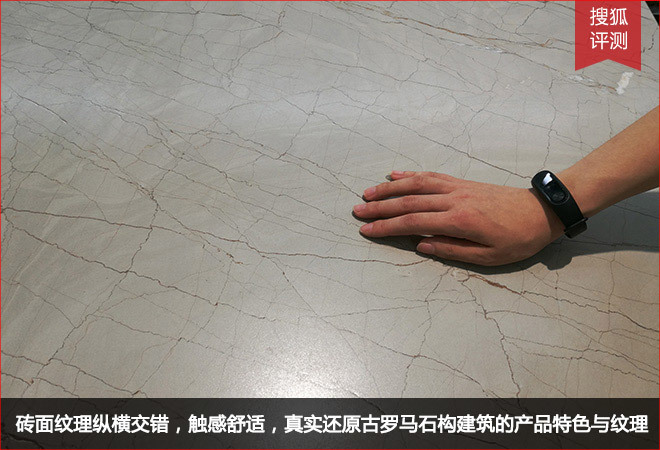为了还原古罗马时代建筑的雄伟气派、历史韵味，法恩莎瓷砖臻稀石3代罗马御石的纹理故意做成纵横交错、自然开裂的感觉，仿佛经历了历史沧桑的古罗马时代建筑，真实还原古罗马石构建筑的产品特色与纹理变化，每一片瓷砖都似乎在诉说着那古老的故事，恢宏大气，尊贵天成。有这样的效果，源于法恩莎瓷砖奢选天然真石为蓝本，进口墨水100%还原真石天然纹理，再加上先进的釉面技术，使得瓷砖纹理深浅不一。另外，小狐抚摸砖面，感觉砖面光滑柔和，犹如婴儿般的细腻肌肤，让人感觉十分舒适，据悉这是法恩莎瓷砖首创Baby Face技术的效果。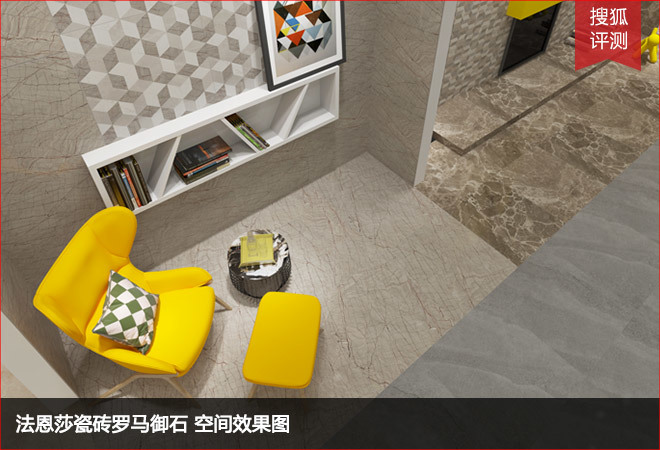在法恩莎瓷砖罗马御石的装饰空间中，我们可以看出罗马御石瓷砖通过设计，加上暖黄色的弧形桌凳、白色造型利落的书架搭配后，整个空间显得既复古又现代感十足，十分符合现代人年轻时尚、简约复古的审美追求。而且罗马御石瓷砖光泽柔和，即使长时间看到这样的瓷砖，眼睛还是十分舒适的。而这得益于法恩莎瓷砖臻稀石3代创新性的使用柔光护瞳技术，光泽度接近天然大理石的光感，呵护视力，关爱家人。小狐对单块砖进行测量可知，它的尺寸是常规的800mm x 800mm，外表与内在呈现出纹理通透的一致性，能够轻松实现不同的拼接效果，让你打造任意的室内设计风格。为了知道瓷砖是否容易铺贴，故特别测试这款瓷砖的平整度，小狐将其立于地面上，看见瓷砖能平稳地“站立”起来，稳固屹立不倒，可知法恩莎罗马御石瓷砖平整度较好。而且其背面纹理呈现整齐的纹格状，更有铺贴方向的提示，有助于砖体的粘合，还能看到背面印有logo作为防伪标识之一，方便消费者选择购买。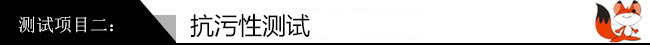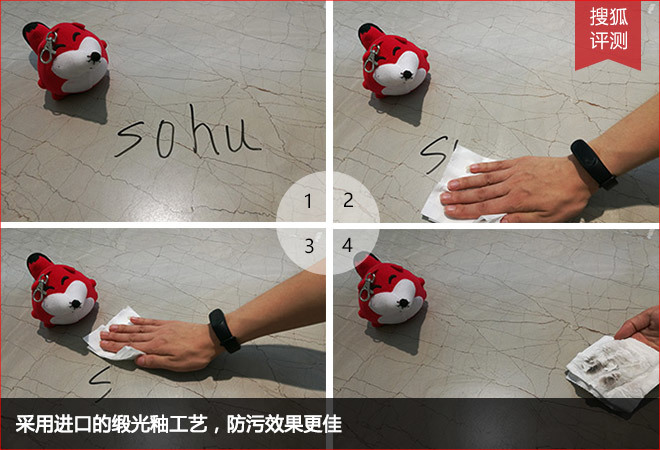卫生间、厨房是家里的卫生重地，尤其是厨房，产生各种餐余垃圾、油烟污渍等，因此需要瓷砖具备较强的防污性能。小狐用油性笔在砖面上写字，静候片刻后，小狐用纸巾就能轻松擦除痕迹，说明这款瓷砖具备较强的防污性能，有利于日清洁打理，能适用于各种不同的空间。据悉，法恩莎瓷砖包括罗马御石在内的臻稀石3代，采用进口的缎光釉工艺，成功突破抛光与防污性互斥、无法兼容的技术瓶颈，防污级别达到五级，效果极佳。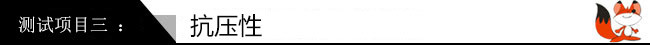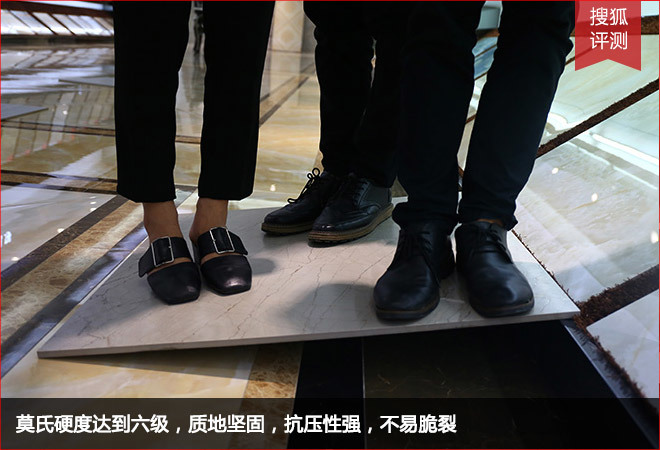小狐邀请3名成年人站立于斜放的砖面上，瓷砖并没有出现弯折、碎裂的迹象，足以说明法恩莎瓷砖罗马御石的硬度与结实度都十分优秀。虽然大理石的主要成分是碳酸钙，质地比较脆，容易断裂，但法恩莎瓷砖通过技术手段不仅逼真还原天然石材的纹理，同时规避了天然石材的弱点，莫氏硬度达到六级，质地坚固，抗压性强，不易脆裂。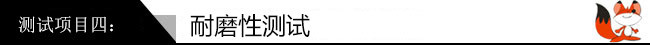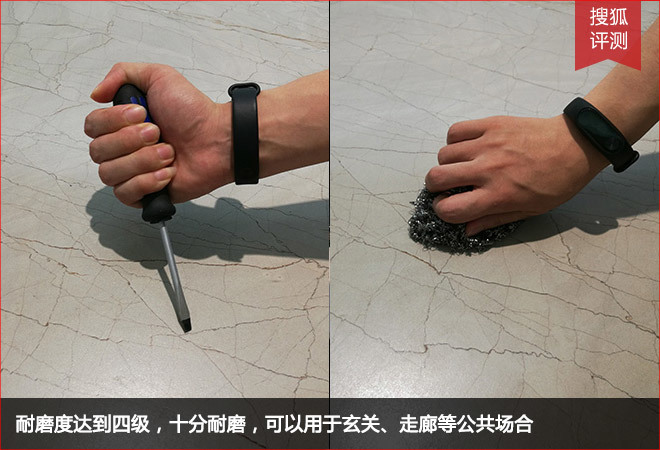当瓷砖作为地板砖使用时，日常走动以及家具摆放等都会对釉面造成一定的磨损，因此要求瓷砖具备较佳的耐磨性能。小狐用钢丝球和螺丝刀刮擦瓷砖表面以测试其是否耐磨，发现瓷砖表面无划痕也无碎屑，多次刮擦之后，瓷砖还是顺滑如初，证明其耐磨性十分好，可以用于玄关、走廊，或是公共场合的使用。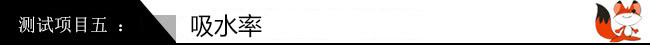吸水率是指石材在标准大气压力下吸水的能力。吸水率越小的石材越紧密坚硬，更禁得起时间的推敲。小狐在瓷砖背面倒上一部分清水，通过10分钟的观察，留意到法恩莎瓷砖罗马御石几乎没有吸水的迹象。随后，小狐用吸水纸吸去水分，砖体背面只留下了淡淡的印迹，由此可以发现，法恩莎瓷砖罗马御石的吸水率是极低的。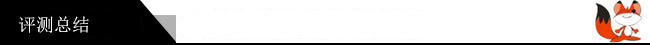法恩莎瓷砖臻稀石3代“罗马御石”，奢选古罗马时代的真石为蓝本，砖面纹理交错，深浅不一，仿佛经过历史变迁自然开裂的古罗马时代建筑，经过设计使空间大气蓬勃、尊贵气派。法恩莎瓷砖采用了多种领先的生产工艺与技术，使其物理性能突出尤其是防污效果极佳，更首创Baby Face技术，让瓷砖呈现婴儿肌肤般的细腻触感，柔光护瞳技术保护眼睛。如果你想倾听古罗马的历史故事，法恩莎瓷砖臻稀石3代“罗马御石”是您的瓷砖首选。拍摄&文字：郭志华

`声明：本文由入驻焦点开放平台的作者撰写，除焦点官方账号外，观点仅代表作者本人，不代表焦点立场错误信息举报电话： 400-099-0099，邮箱：jubao@vip.sohu.com，或点此进行意见反馈，或点此进行举报投诉。`A B C D E F G H J K L M N P Q R S T W X Y Z
A - B - C - D - E
• A
• 鞍山
• 安庆
• 安阳
• 安顺
• 安康
• 澳门
• B
• 北京
• 保定
• 包头
• 巴彦淖尔
• 本溪
• 蚌埠
• 亳州
• 滨州
• 北海
• 百色
• 巴中
• 毕节
• 保山
• 宝鸡
• 白银
• 巴州
• C
• 承德
• 沧州
• 长治
• 赤峰
• 朝阳
• 长春
• 常州
• 滁州
• 池州
• 长沙
• 常德
• 郴州
• 潮州
• 崇左
• 重庆
• 成都
• 楚雄
• 昌都
• 慈溪
• 常熟
• D
• 大同
• 大连
• 丹东
• 大庆
• 东营
• 德州
• 东莞
• 德阳
• 达州
• 大理
• 德宏
• 定西
• 儋州
• 东平
• E
• 鄂尔多斯
• 鄂州
• 恩施
F - G - H - I - J
• F
• 抚顺
• 阜新
• 阜阳
• 福州
• 抚州
• 佛山
• 防城港
• G
• 赣州
• 广州
• 桂林
• 贵港
• 广元
• 广安
• 贵阳
• 固原
• H
• 邯郸
• 衡水
• 呼和浩特
• 呼伦贝尔
• 葫芦岛
• 哈尔滨
• 黑河
• 淮安
• 杭州
• 湖州
• 合肥
• 淮南
• 淮北
• 黄山
• 菏泽
• 鹤壁
• 黄石
• 黄冈
• 衡阳
• 怀化
• 惠州
• 河源
• 贺州
• 河池
• 海口
• 红河
• 汉中
• 海东
• I
• J
• 晋中
• 锦州
• 吉林
• 鸡西
• 佳木斯
• 嘉兴
• 金华
• 景德镇
• 九江
• 吉安
• 济南
• 济宁
• 焦作
• 荆门
• 荆州
• 江门
• 揭阳
• 金昌
• 酒泉
• 嘉峪关
K - L - M - N - P
• K
• 开封
• 昆明
• 昆山
• L
• 廊坊
• 临汾
• 辽阳
• 连云港
• 丽水
• 六安
• 龙岩
• 莱芜
• 临沂
• 聊城
• 洛阳
• 漯河
• 娄底
• 柳州
• 来宾
• 泸州
• 乐山
• 六盘水
• 丽江
• 临沧
• 拉萨
• 林芝
• 兰州
• 陇南
• M
• 牡丹江
• 马鞍山
• 茂名
• 梅州
• 绵阳
• 眉山
• N
• 南京
• 南通
• 宁波
• 南平
• 宁德
• 南昌
• 南阳
• 南宁
• 内江
• 南充
• P
• 盘锦
• 莆田
• 平顶山
• 濮阳
• 攀枝花
• 普洱
• 平凉
Q - R - S - T - W
• Q
• 秦皇岛
• 齐齐哈尔
• 衢州
• 泉州
• 青岛
• 清远
• 钦州
• 黔南
• 曲靖
• 庆阳
• R
• 日照
• 日喀则
• S
• 石家庄
• 沈阳
• 双鸭山
• 绥化
• 上海
• 苏州
• 宿迁
• 绍兴
• 宿州
• 三明
• 上饶
• 三门峡
• 商丘
• 十堰
• 随州
• 邵阳
• 韶关
• 深圳
• 汕头
• 汕尾
• 三亚
• 三沙
• 遂宁
• 山南
• 商洛
• 石嘴山
• T
• 天津
• 唐山
• 太原
• 通辽
• 铁岭
• 泰州
• 台州
• 铜陵
• 泰安
• 铜仁
• 铜川
• 天水
• 天门
• W
• 乌海
• 乌兰察布
• 无锡
• 温州
• 芜湖
• 潍坊
• 威海
• 武汉
• 梧州
• 渭南
• 武威
• 吴忠
• 乌鲁木齐
X - Y - Z
• X
• 邢台
• 徐州
• 宣城
• 厦门
• 新乡
• 许昌
• 信阳
• 襄阳
• 孝感
• 咸宁
• 湘潭
• 湘西
• 西双版纳
• 西安
• 咸阳
• 西宁
• 仙桃
• 西昌
• Y
• 运城
• 营口
• 盐城
• 扬州
• 鹰潭
• 宜春
• 烟台
• 宜昌
• 岳阳
• 益阳
• 永州
• 阳江
• 云浮
• 玉林
• 宜宾
• 雅安
• 玉溪
• 延安
• 榆林
• 银川
• Z
• 张家口
• 镇江
• 舟山
• 漳州
• 淄博
• 枣庄
• 郑州
• 周口
• 驻马店
• 株洲
• 张家界
• 珠海
• 湛江
• 肇庆
• 中山
• 自贡
• 资阳
• 遵义
• 昭通
• 张掖
• 中卫

1室1厅1厨1卫1阳台

1
2
3
4
5

0
1
2

1

1

0
1
2
3报名成功，资料已提交审核A B C D E F G H J K L M N P Q R S T W X Y Z
A - B - C - D - E
• A
• 鞍山
• 安庆
• 安阳
• 安顺
• 安康
• 澳门
• B
• 北京
• 保定
• 包头
• 巴彦淖尔
• 本溪
• 蚌埠
• 亳州
• 滨州
• 北海
• 百色
• 巴中
• 毕节
• 保山
• 宝鸡
• 白银
• 巴州
• C
• 承德
• 沧州
• 长治
• 赤峰
• 朝阳
• 长春
• 常州
• 滁州
• 池州
• 长沙
• 常德
• 郴州
• 潮州
• 崇左
• 重庆
• 成都
• 楚雄
• 昌都
• 慈溪
• 常熟
• D
• 大同
• 大连
• 丹东
• 大庆
• 东营
• 德州
• 东莞
• 德阳
• 达州
• 大理
• 德宏
• 定西
• 儋州
• 东平
• E
• 鄂尔多斯
• 鄂州
• 恩施
F - G - H - I - J
• F
• 抚顺
• 阜新
• 阜阳
• 福州
• 抚州
• 佛山
• 防城港
• G
• 赣州
• 广州
• 桂林
• 贵港
• 广元
• 广安
• 贵阳
• 固原
• H
• 邯郸
• 衡水
• 呼和浩特
• 呼伦贝尔
• 葫芦岛
• 哈尔滨
• 黑河
• 淮安
• 杭州
• 湖州
• 合肥
• 淮南
• 淮北
• 黄山
• 菏泽
• 鹤壁
• 黄石
• 黄冈
• 衡阳
• 怀化
• 惠州
• 河源
• 贺州
• 河池
• 海口
• 红河
• 汉中
• 海东
• I
• J
• 晋中
• 锦州
• 吉林
• 鸡西
• 佳木斯
• 嘉兴
• 金华
• 景德镇
• 九江
• 吉安
• 济南
• 济宁
• 焦作
• 荆门
• 荆州
• 江门
• 揭阳
• 金昌
• 酒泉
• 嘉峪关
K - L - M - N - P
• K
• 开封
• 昆明
• 昆山
• L
• 廊坊
• 临汾
• 辽阳
• 连云港
• 丽水
• 六安
• 龙岩
• 莱芜
• 临沂
• 聊城
• 洛阳
• 漯河
• 娄底
• 柳州
• 来宾
• 泸州
• 乐山
• 六盘水
• 丽江
• 临沧
• 拉萨
• 林芝
• 兰州
• 陇南
• M
• 牡丹江
• 马鞍山
• 茂名
• 梅州
• 绵阳
• 眉山
• N
• 南京
• 南通
• 宁波
• 南平
• 宁德
• 南昌
• 南阳
• 南宁
• 内江
• 南充
• P
• 盘锦
• 莆田
• 平顶山
• 濮阳
• 攀枝花
• 普洱
• 平凉
Q - R - S - T - W
• Q
• 秦皇岛
• 齐齐哈尔
• 衢州
• 泉州
• 青岛
• 清远
• 钦州
• 黔南
• 曲靖
• 庆阳
• R
• 日照
• 日喀则
• S
• 石家庄
• 沈阳
• 双鸭山
• 绥化
• 上海
• 苏州
• 宿迁
• 绍兴
• 宿州
• 三明
• 上饶
• 三门峡
• 商丘
• 十堰
• 随州
• 邵阳
• 韶关
• 深圳
• 汕头
• 汕尾
• 三亚
• 三沙
• 遂宁
• 山南
• 商洛
• 石嘴山
• T
• 天津
• 唐山
• 太原
• 通辽
• 铁岭
• 泰州
• 台州
• 铜陵
• 泰安
• 铜仁
• 铜川
• 天水
• 天门
• W
• 乌海
• 乌兰察布
• 无锡
• 温州
• 芜湖
• 潍坊
• 威海
• 武汉
• 梧州
• 渭南
• 武威
• 吴忠
• 乌鲁木齐
X - Y - Z
• X
• 邢台
• 徐州
• 宣城
• 厦门
• 新乡
• 许昌
• 信阳
• 襄阳
• 孝感
• 咸宁
• 湘潭
• 湘西
• 西双版纳
• 西安
• 咸阳
• 西宁
• 仙桃
• 西昌
• Y
• 运城
• 营口
• 盐城
• 扬州
• 鹰潭
• 宜春
• 烟台
• 宜昌
• 岳阳
• 益阳
• 永州
• 阳江
• 云浮
• 玉林
• 宜宾
• 雅安
• 玉溪
• 延安
• 榆林
• 银川
• Z
• 张家口
• 镇江
• 舟山
• 漳州
• 淄博
• 枣庄
• 郑州
• 周口
• 驻马店
• 株洲
• 张家界
• 珠海
• 湛江
• 肇庆
• 中山
• 自贡
• 资阳
• 遵义
• 昭通
• 张掖
• 中卫• 手机• 分享
• 设计
免费设计
• 计算器
装修计算器
• 入驻
合作入驻
• 联系
联系我们
• 置顶
返回顶部